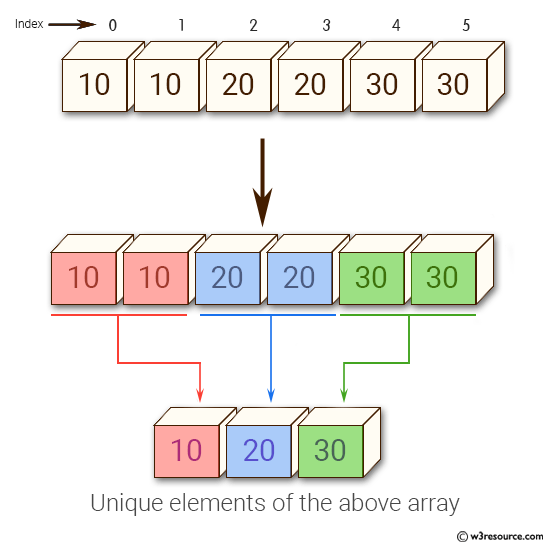﻿ NumPy: Get the unique elements of an array - w3resource# NumPy: Get the unique elements of an array

## NumPy: Array Object Exercise-19 with Solution

Write a NumPy program to get the unique elements of an array.

Pictorial Presentation:Sample Solution:-

Python Code:

``````import numpy as np
x = np.array([10, 10, 20, 20, 30, 30])
print("Original array:")
print(x)
print("Unique elements of the above array:")
print(np.unique(x))
x = np.array([[1, 1], [2, 3]])
print("Original array:")
print(x)
print("Unique elements of the above array:")
print(np.unique(x))
```
```

Sample Output:

```Original array:
[10 10 20 20 30 30]
Unique elements of the above array:
[10 20 30]
Original array:
[[1 1]
[2 3]]
Unique elements of the above array:
[1 2 3]
```

Python Code Editor:

Have another way to solve this solution? Contribute your code (and comments) through Disqus.

What is the difficulty level of this exercise?

Test your Python skills with w3resource's quiz

﻿

## Python: Tips of the Day

Set comprehension:

```>>> m = {x ** 2 for x in range(5)}
>>> m
{0, 1, 4, 9, 16}
```